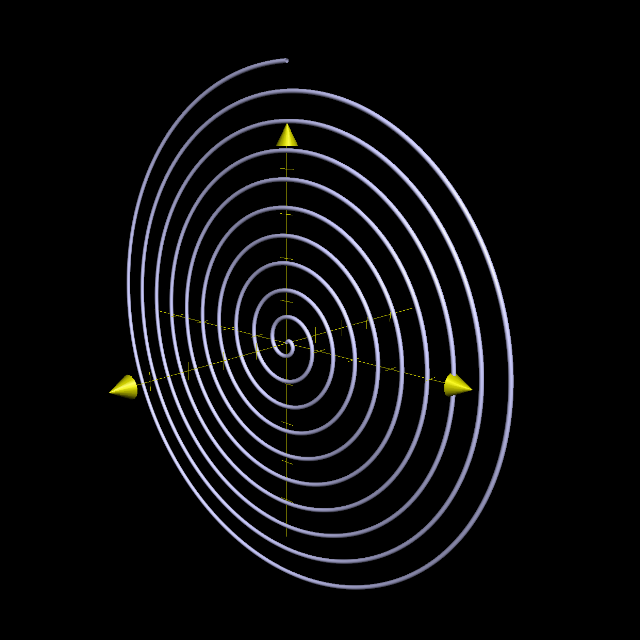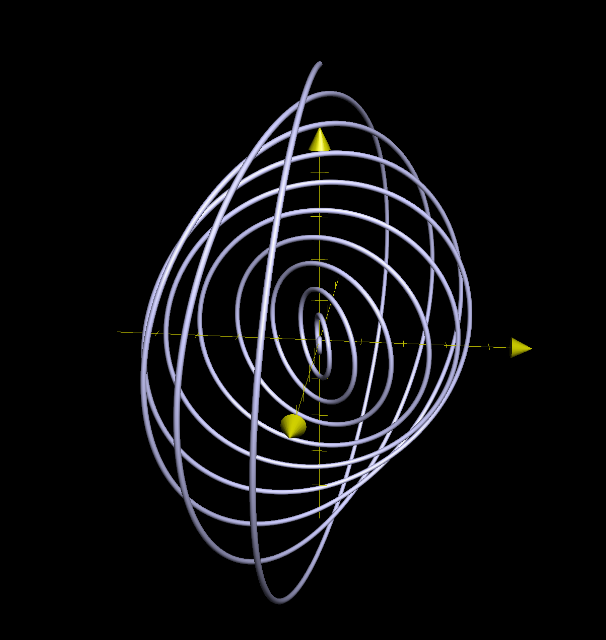## Wednesday, May 18, 2016

### A Calculation in Statistical Mechanics

We've been harping about phase and state space for a long while, now, and have mentioned at more than one point that statistical mechanics is a subject dealing with, in some sense, the ultimate state space, of many gazillions of dimensions. It is amazing that we can even calculate anything about such spaces. So we take a break from tori, and return to the (music of the) spheres, today, to give an example. We consider $N$-dimensional spheres, for $N = 0, 1, 2, 3, \dots$ and their "volumes". Note, of course, by $N$-sphere, we always mean the curved surface of points in $(N+1)$-dimensional space with unit distance from the orgin, and not the whole ball. [The $3$-sphere, as we know, is foliated by nested tori. We aren't going TOO far afield].

To talk about this right, we need a little physics. Most of you probably have heard of the principle of conservation of energy... If you haven't, or even if you have, make sure to read about how Hamiltonian Mechanics is Awesome. Anyway, so if you imagine little billiard balls of uniform mass $m$ freely zipping about, banging into stuff, each particle having position $\mathbf{q}_i$ and momentum $\mathbf{p}_i$, then since we know Hamiltonian Mechanics is Awesome, this says that the two quantities evolve in such a way that the energy of the $i$th particle $E_i = \frac{|\mathbf{p}_i|^2}{2m}$
stays constant. But if you imagine having a very, very large number of such billiard balls (several billiards of such (1 billiard = $10^{15}$ in most dialects of English, or such equivalent cognates) HAR HAR!), and compute the total energy, summing them all up,$H = \sum_i E_i = \sum_i \frac{|\mathbf{p}_i|^2}{2m}.$ To say that the total energy is constant, then, is to say $\sum_i \frac{|\mathbf{p}_i|^2}{2m} = E$ for some fixed number $E$. But what does this say? If we write out each $|\mathbf{p}_i|^2 = p_{ix}^2 + p_{iy}^2 + p_{iz}^2,$ then $2mE=\sum_i p_{ix}^2 + p_{iy}^2 + p_{iz}^2,$ meaning that if we sum over all the particles, say $N$ of them, where $N \approx 10^{24}$ (a milliard billiard of billiard balls), this means we're summing the squares of a $3N$-dimensional vector and equating it to a constant. So if we ask ourselves:

What is the collection of all possible momenta of $N$ particles that have one constant energy level $E$,

this is the same as saying,

What are all the points on the $(3N-1)$-dimensional sphere of radius $\sqrt{2mE}$?

(the -1 from the fact that you're expressing the surface as the solution set to one equation: the boundary of the $k$-ball is the $(k-1)$-sphere) Interesting, you might say, but is there any occasion which actually requires you to truly do calculations treating it precisely as a geometrical $(3N-1)$-dimensional object?

Well, what's one of the first things you think about when talking geometry? Areas and volumes, of course. There are situations in which you need to integrate over such energy "surface", or within the $N$-ball contained within, for $N \sim 10^{24}$. Basically, to find quantities like entropy, one needs to "count some number of cells" inside the $3N$-ball . This yields the total "number" of energy states with level less than or equal to $E$. "Number" in quotations because classically, of course, the actual number of states is the number of points on a sphere, which is of course uncountably infinite. We instead mean, per unit $3N$-dimensional volume, which actually leads to the density of states. The distinction is a little blurry, because, with a little help of quantum mechanics, we can think of each state as a cell of volume $(2\pi \hbar)^{3N}$, and each distinct state of momentum, which must be quantized, ultimately arises from boundary conditions in solving Schrödinger's equation, or eigenstuff.

Suffice to say, however, let's talk as if calculating the volume of the $3N$-ball does indeed count the total number of momentum states with energy less than or equal to $E$, i.e., let's calculate $\frac{1}{(2\pi \hbar)^{3N}}\int_{|\mathbf{p}|^2 \leq 2mE} d\mathbf{q}d\mathbf{p} =\frac{V^N}{(2\pi \hbar)^{3N}}\int_{|\mathbf{p}|^2 \leq 2mE} d\mathbf{p} .$
where $V$ is the ordinary 3D volume of the spatial region where all our particles live. For the momentum integral, we need the formula (whose derivation is fodder for another post!) for the volume of an $K$-ball $V_K(R) = \frac{\pi^{K/2}}{\Gamma\left(1+\frac{K}{2}\right)}R^K$
where $\Gamma(1+ K/2)$ is $(K/2)!$, the factorial of $K/2$, if $K$ is even, and $\pi^{1/2} K!!/2^{K/2}$ where $K!!$ is the double factorial $1\cdot 3 \cdot 5 \cdots k$, for $k$ odd.

Plugging this in with $R = \sqrt{2mE}$ and $K=3N$ we have the total volume of a $3N$-ball in momentum space: $V_{3N}(\sqrt{2mE}) = \frac{\pi^{3N/2}}{\Gamma\left(1+\frac{3N}{2}\right)} (2mE)^{3N/2}$
To make headway here, we can use Stirling's Approximation for the Gamma function:$\Gamma\left(1+\frac{3N}{2}\right) \approx \sqrt{3N\pi} \left(\frac{3N}{2e}\right)^{3N/2}$ This gives $V_{3N}(\sqrt{2mE}) \approx \frac{1}{\sqrt{3\pi N}}\left(\frac{4\pi meE}{3N} \right)^{3N/2}$ or, the total number of states is $\#\text{states} = \frac{V^N V_{3N}(\sqrt{2mE})}{(2\pi \hbar)^{3N}} \approx \frac{1}{\sqrt{3\pi N}}\left(\frac{4\pi meEV^{2/3}}{3N (2\pi \hbar)^2} \right)^{3N/2}$
Considering the smallness of $\hbar$ ($10^{-34}$ in everyday units) and the exponent $3N/2$ being on the order of $10^{24}$, this could be a staggeringly huge number (but that's what you expect: for lots of particles, they really can occupy even more states. For example, two particles behaving independently is the square the total number of states of for one particle). Thus to measure entropy, we take the natural log of all of this (and add a factor of the Boltzmann constant, $k$ which is on the order of $10^{-23}$ in ordinary units), we get $k\ln(\#\text{states}) = \frac{3Nk}{2} \left( \ln \left(\frac{4\pi mEV^{2/3}}{3N(2\pi \hbar)^2}\right) +1\right) -\frac{1}{2}\ln(3\pi N)k.$
Since those last terms are small potatoes (not being multiplied by $N$), we usually just drop it to arrive at the entropy of our system of particles as
$S = \frac{3Nk}{2} \left( \ln \left(\frac{4\pi mEV^{2/3}}{3N(2\pi \hbar)^2}\right)\right)+\frac{3Nk}{2}.$ And for you chemists, $Nk = nR$, where $n$ is the number of moles and $R$ is the gas constant:
$S = \frac{3nR}{2}\left( \ln \left(\frac{4\pi mEV^{2/3}}{3N(2\pi \hbar)^2}\right)\right)+\frac{3nR}{2}.$
That's really nasty. How about we try to plug numbers into this and see what it is? We'll do that more concretely next time. But suffice to say, let's get back to what's interesting: we calculated the volume of some high-dimensional stuff!

## Friday, May 13, 2016

### The Volume of Intersecting Cylinders

Now for another classic, although not as "kewel" as the spiral example. This time, it was I who asked for the help: once upon a time in a calculus class, we were asked to compute the volume of the solid that is the intersection of two cylinders at right angles. This is a surprisingly subtle calculation, and one of the more difficult-to-visualize things. Fortunately, I've managed to reproduce it above, with some transparency so the intersection is a little bit more visible. Now, here is the full intersection itself without the extra cylinder parts (called the Steinmetz Solid):

It is said that the Chinese mathematician 祖沖之 (Zu Chongzhi) computed the volume of this solid in the 400s ... I don't know how he did it without calculus, which is the way we're going to do it today. To do this, we write the equations of the two cylinders, say of radius $R$, which we will assume to have their axes in the $xy$-plane:
$x^2 + z^2 = R^2$ $y^2 + z^2 = R^2.$
Let's see what the actual intersection is. We set the two equations equal to each other:
$x^2 + z^2 = y^2 +z^2$
which means $x^2 = y^2$. This in turn means $y = \pm x$. In the $xy$-plane, this looks like two intersecting lines in an "X" shape, which you can see by looking at the solid above from the very tippy top. Of course, this is not exactly what the intersection actually looks like: we still have that there is a $z$-coordinate, given by $z = \pm \sqrt{R^2-x^2} =\pm \sqrt{R^2-y^2}$, so that the complete parametrization of the curves (four of them corresponding to each choice of $+$ or $-$) are given by, for example
$x = t$$y = \pm t$$z = \pm\sqrt{R^2-t^2}$
for $t$ varying from $-R$ to $R$ (the maximum allowable range given that it has to lie on a cylinder). This gives the "corners" of the solid given above. The solid itself is given by stacking squares with edges running from one curve to another (you can also see that in the solid above). To calculate the volume, we integrate the area of cross-sections perpendicular to the $z$-axis:
$V=\int_{-R}^R A(z)\,dz = \int_{-R}^R s^2\,dz.$
Therefore, we must find the side length $s$, which requires us to solve things in terms of $z$: $z =\pm\sqrt{R^2-x^2}$ means $z^2 = R^2 - x^2$, or $x^2 = R^2 - z^2$, so finally $x = \pm\sqrt{R^2 - z^2}$. Since the sides of the square are the same on either side, we can choose the positive square root. Then, since one side of the square goes between the two different values of $y$ for a given $x$, we find $s = \sqrt{R^2 - z^2}-(-\sqrt{R^2 - z^2}) = 2\sqrt{R^2 - z^2}$. Finally, we can find the integral:
$V = \int_{-R}^R s^2\, dz = \int_{-R}^{R} 4(R^2-z^2)\,dz = \left.4R^2 z - \frac{4}{3}z^3\right|_{-R}^R = \left(8-\frac{8}{3}\right)R^3 = \frac{16}{3}R^3.$

## Wednesday, May 11, 2016

### Yitz..'s Kewel Spiral

Every once in a while, I get an "assignment" to calculate some interesting parametrization (because parametrizations are awesome like that). For example, the bowtie pasta was originally a request from a student. Today's goes way back, to someone asking a question on a forum in math way back when. He needed a spiral that started out and twisted its way through space as it got larger. In essence, a spiral that doesn't stay in the same plane. Obviously, polar curves are out of the question, since they are, well, in the plane. But what's one dimension up from polar coordinates? Spherical coordinates $(r,\varphi,\theta)$, of course (or if you're a physicist, $(r,\theta,\phi)$).We fall back on a good ol' tool, parametrization.

To discover what its equation might be, we first consider an Archimedean spiral ($r = a\varphi$) in the $r\varphi$-plane (or $yz$-plane), or possibly a logarithmic one, like $e^{\varphi/3}$, which grows as it goes around (like some spirals last time) the "pole" which is now the $x$-axis. This ends up growing as you move clockwise, rather than counterclockwise, since $\varphi$ increases as it goes from north toward east.

This is most efficiently represented in a parametric manner: first, in the $yz$-plane:$x=0,$ $y = at \sin t,$ $z = at \cos t.$
gives the parametric equation of the Archimedean spiral in the $yz$-plane going clockwise. This gives $\varphi = t$ and $r = at$, which recovers the original polar equation $r = a\varphi$.Archimedean Spiral in the $yz$-plane, with $a = 1/20$.

How do we give it a twist? We reason that, after each revolution, we want it twisted out of the plane by some small amount (say, rotated about the $z$-axis by $b$ times a full revolution): we apply the rotation to the $x$ and $y$, giving:$x=at\sin t \cos (bt),$ $y = at \sin t \sin(bt),$ $z = at \cos t.$Twisting out of the plane with parameter $b = 1/20$, also.

Here's the original one I delivered to Yitz.. (it is actually exponential):
Pretty kewel, right? (that was his exact word to describe it).

1 I always use the convention that if I indeed need to refer to a physics text, do calculations to be consistent... but I always write my $\phi$'s as non-curly in that context... I haven't yet figured how to draw my $\theta$s different though. Yes, I know there is $\vartheta$, but it hasn't caught on in my notation. It just doesn't feel enough like a $\theta$.